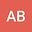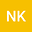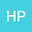On fundamental group of soft topological spaces
•••• Ali Akbar Bahredar,
Ali Akbar Bahredar
University of Sistan and Baluchestan
Author ProfileIn this paper we introduce the notion of fundamental group for soft topological spaces. To do so, we define soft paths, soft loops and the notion of $$\xi$$-soft path homotopy, and study some of their basic properties. We also show that the fundamental group of an $$\varepsilon$$-soft topological group is commutative, and that $$\pi_{1}^{soft}$$ is a functor between the category of soft topological spaces and the category of groups.